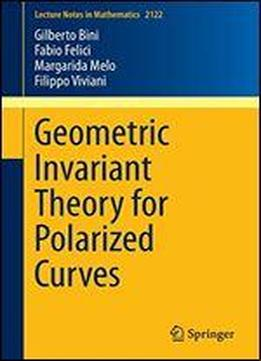# Geometric Invariant Theory For Polarized Curves (lecture Notes In Mathematics) by Fabio Felici / 2014 / English / PDF

We investigate GIT quotients of polarized curves. More specifically, we study the GIT problem for the Hilbert and Chow schemes of curves of degree d and genus g in a projective space of dimension d-g, as d decreases with respect to g. We prove that the first three values of d at which the GIT quotients change are given by d=a(2g-2) where a=2, 3.5, 4. We show that, for a4, L. Caporaso's results hold true for both Hilbert and Chow semistability. If 3.5a4, the Hilbert semistable locus coincides with the Chow semistable locus and it maps to the moduli stack of weakly-pseudo-stable curves. If 2a3.5, the Hilbert and Chow semistable loci coincide and they map to the moduli stack of pseudo-stable curves. We also analyze in detail the critical values a=3.5 and a=4, where the Hilbert semistable locus is strictly smaller than the Chow semistable locus. As an application, we obtain three compactications of the universal Jacobian over the moduli space of stable curves, weakly-pseudo-stable curves and pseudo-stable curves, respectively.

views: 239